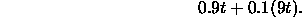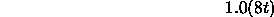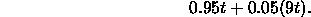# Homework 1 Solution

CS26

1. 1.4. Submit a diagram showing the program executions (similar to Figure 1.5) along with your calculations.

Part a. A non-overlapped execution needs 19 time units. For a long sequence of programs, each overlapped execution needs 16 time units (it gets three units for ``free'' because of the overlap). The ratio is 16/19.

Part b. Let the non-overlapped time for a single execution be t. For a long sequence of programs, each overlapped execution can overlap it input with the previous program's computing and can overlap its computing with the previous program's output. So, it gets 2 t/3 for ``free.'' The ratio is 1/3.

2. 1.6.

Part a. Let the cache access time be t. The execution time with a cache is proportional toThe first time is the contribution from cache hits, while the second is the contribution from cache misses. The time is 9 t because the memory access loads the cache and then the cache is used to load the instruction register.

The execution time without a cache is proportional toThe ratio is 4.44.

Part b. Let the cache access time be t. The execution time with a cache is proportional toThe execution time without a cache is still proportional toThe ratio is 5.71. What's important to note here is that doubling the size of the cache didn't halve the execution time.

3. 2.5.

Following the text's example, which implies that the accumulator is the only register:

```            load A
mult B
store TEMP
mult D
store RESULT
```

4. 2.7, 2.8. Note that A and B are arrays.

2.7.

```            load N,r0            // 2
load #A,r1           // 2  pointer into A
load #b,r2           // 2  pointer into B
load #0,r3           // 1  accumulated sum of products

loop:       branch r0==0,eloop   // 1
load (r1)+,r4        // 2  get A[i]
load (r2)+,r5        // 2  get B[i]
mult r4,r5           // 1
branch loop          // 1

eloop:      store r3,C           // 2
```
The number in each comment is the number of memory accesses for the instruction. Note that the branch at the top of the loop is executed one extra time. The equation is 10 + 9n.

2.8

```            load N,r0            // 2

loop:       branch r0==0,eloop   // 1
mult (r2)+,r4        // 2
branch loop          // 1

eloop:      store r3,C           // 2
```
The equation is 10 + 8n. We save one memory access in the loop by being able to combine a load and multiply. I continue to use several registers because of the speed disparity between a CPU and memory.

5. 2.10.

1220, 3000, 5830, 4599 (pre-decrement), 1200 (post-increment).

6. 2.17.

b and c support subroutine nesting. Only c supports recursion. Because the calls are all from the same place, b would scribble over the previous return address with the next return address.

7. 2.18.

Store the link register's value on a stack at the start of a subroutine. This would permit recursion.

Thomas P. Kelliher
Thu Sep 26 12:19:49 EDT 1996
Tom Kelliher